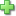# Math-U-See Algebra 1 - Student Pack

Publisher: Math-U-See
Legacy Edition, ©2009, Publisher Catalog #1111
Hardcover
Price: \$48.00

See series description for full review.

# Algebra 1

### Graphing, Simultaneous Equations, Exponents, Polynomials, Unit Multipliers, and More

Algebra 1 teaches all basic algebra concepts. These include basic properties, solving equations (including simultaneous equations), coin problems, exponents and square roots, scientific notation, unit multipliers, and metric conversions. Graphing lines is taught in detail, and graphing conic sections is introduced. Operations with polynomials are demonstrated with place-value blocks for conceptual understanding before being taught conventionally.

## Student Pack

For customers who already have the needed Integer Block Kit, Algebra/Decimal Insert Kit, and instruction materials.

The Student Pack includes:

• Student Workbook
• Tests booklet

Each student will need their own Student Workbook and Tests booklet.

Scope and Sequence:

• Lesson 01: Commutative and Associative Properties
• Lesson 02: Order of Operations and Absolute Value
• Lesson 03: Solving for an Unknown with One Variable
• Lesson 04: Distributive Property
• Lesson 05: Number Lines and Cartesian Coordinates
• Lesson 06: Graphing a Line
• Lesson 07: Slope-Intercept Formula
• Lesson 08: Graphing a Line from the Slope-Intercept Formula
• Lesson 09: Graphing Parallel Lines and the Standard Equation of a Line
• Lesson 10: Graphing Perpendicular Lines
• Lesson 11: Finding the Slope-Intercept Formula with Different Givens
• Lesson 12: Graphing Inequalities
• Lesson 13: Solving Simultaneous Equations by Graphing
• Lesson 14: Solving Simultaneous Equations by Substitution
• Lesson 15: Solving Simultaneous Equations by Elimination
• Lesson 16: Coin Problems
• Lesson 17: Consecutive Integers
• Lesson 18: Multiplication and Division with Exponents
• Lesson 19: Negative Exponents and Raising Exponents to a Power
• Lesson 20: Addition and Multiplication of Polynomials
• Lesson 21: Factor Polynomials
• Lesson 22: Factor Trinomials with Coefficients
• Lesson 23: Factor Trinomials with Negative Numbers
• Lesson 24: Square Roots and Dividing Polynomials
• Lesson 25: Difference of Two Squares and Oriental Squares
• Lesson 26: Repeated Factoring of Polynomials
• Lesson 27: Solving Equations with Factoring
• Lesson 28: Unit Multipliers
• Lesson 29: Square Unit Multipliers
• Lesson 30: Metric Conversions
• Lesson 31: Fractional Exponents
• Lesson 32: Significant Digits and Scientific Notation
• Lesson 33: Bases Other Than Ten
• Lesson 34: Graphing a Circle and an Ellipse
• Lesson 35: Graphing a Parabola and a Hyperbola

## Sample Lesson

Did you find this review helpful?
Series Description
Related CategoriesClick here to write a review